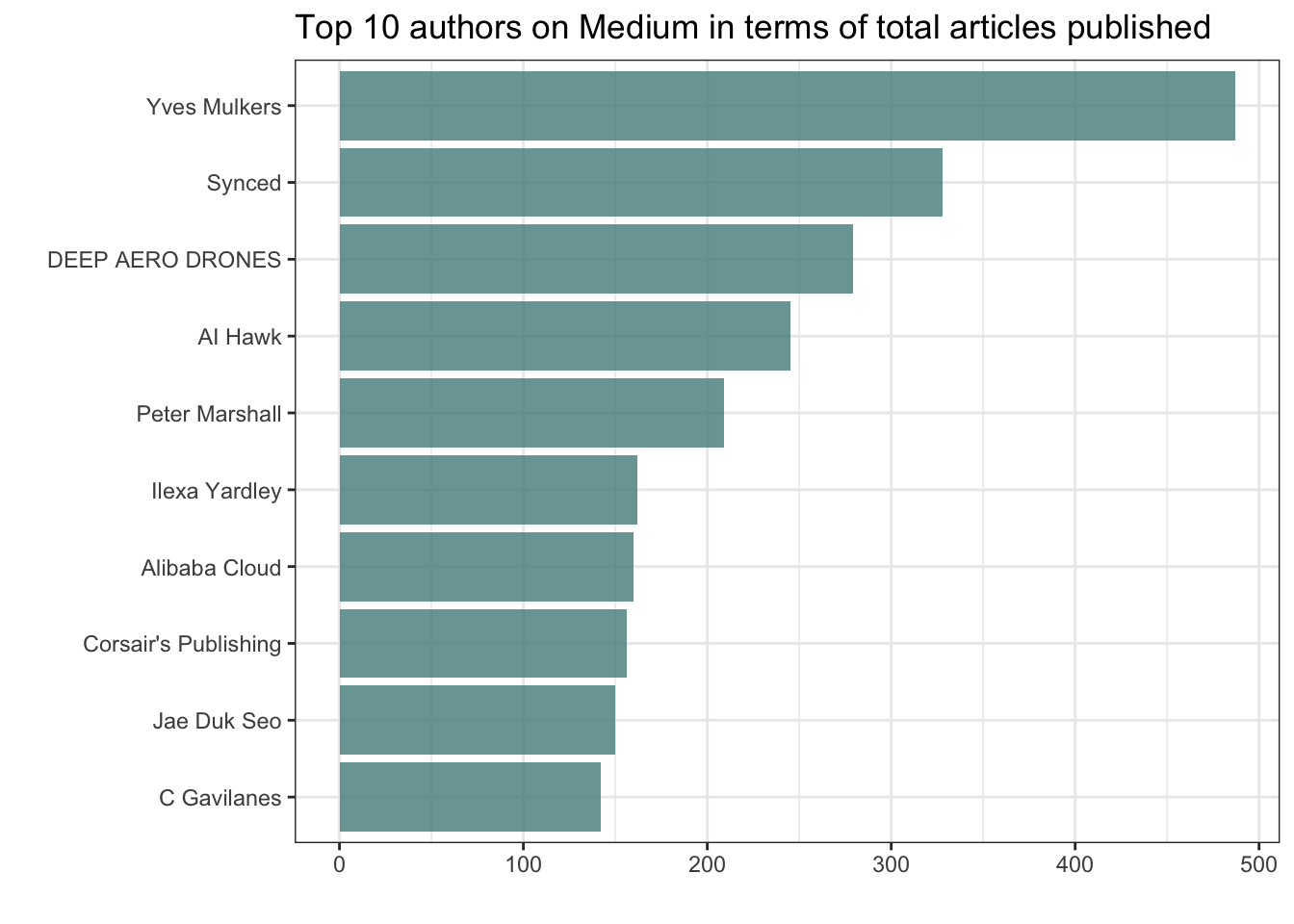Analyzing data for #tidytuesday week of 12/4/2018 (source)

``````# LOAD PACKAGES AND PARSE DATA
library(tidyverse)
library(scales)
library(RColorBrewer)
library(forcats)
library(tidytext)
library(stringr)

articles <- articles_raw``````

### Who are the top 10 authors in terms of total articles published?

``````top_authors <- articles %>%
select(author) %>%
group_by(author) %>%
count() %>%
arrange(desc(n)) %>%
na.omit() %>%

top_authors %>%
ggplot() +
geom_col(aes(reorder(author, n), n),
fill = "darkslategray4",
alpha = 0.8) +
coord_flip() +
theme_bw() +
labs(x = "",
y = "",
title = "Top 10 authors on Medium in terms of total articles published")``````### Are there differences in words used between the titles and subtitles?

Clean up data before we text mine the top 10 authors

``````data(stop_words)

tidy_authors <-
articles %>%
inner_join(top_authors) %>%
select(title, subtitle, author) %>%
na.omit() %>%
mutate(text = paste(title, " ", subtitle)) %>%
select(author, text) %>%
unnest_tokens(word, text) %>%
anti_join(stop_words)``````

Calculate proportions and plot graph

``````tidy_authors %>%
group_by(author) %>%
mutate(word = str_extract(word, "[a-z']+")) %>%
count(word, sort = TRUE) %>%
mutate(proportion = n / sum(n)) %>%
select(-n) %>%
gather(author, proportion, `AI Hawk`:`Synced`) %>%
ggplot(aes(x=proportion, y=`Yves Mulkers`, color = abs(`Yves Mulkers` - proportion))) +
geom_jitter(alpha = 0.1,
size = 0.5,
width = 0.25,
height = 0.25) +
geom_text(aes(label = word),
check_overlap = TRUE,
vjust = 1,
hjust = 1) +
geom_abline(color = "darkslategray4",
linetype = 2) +